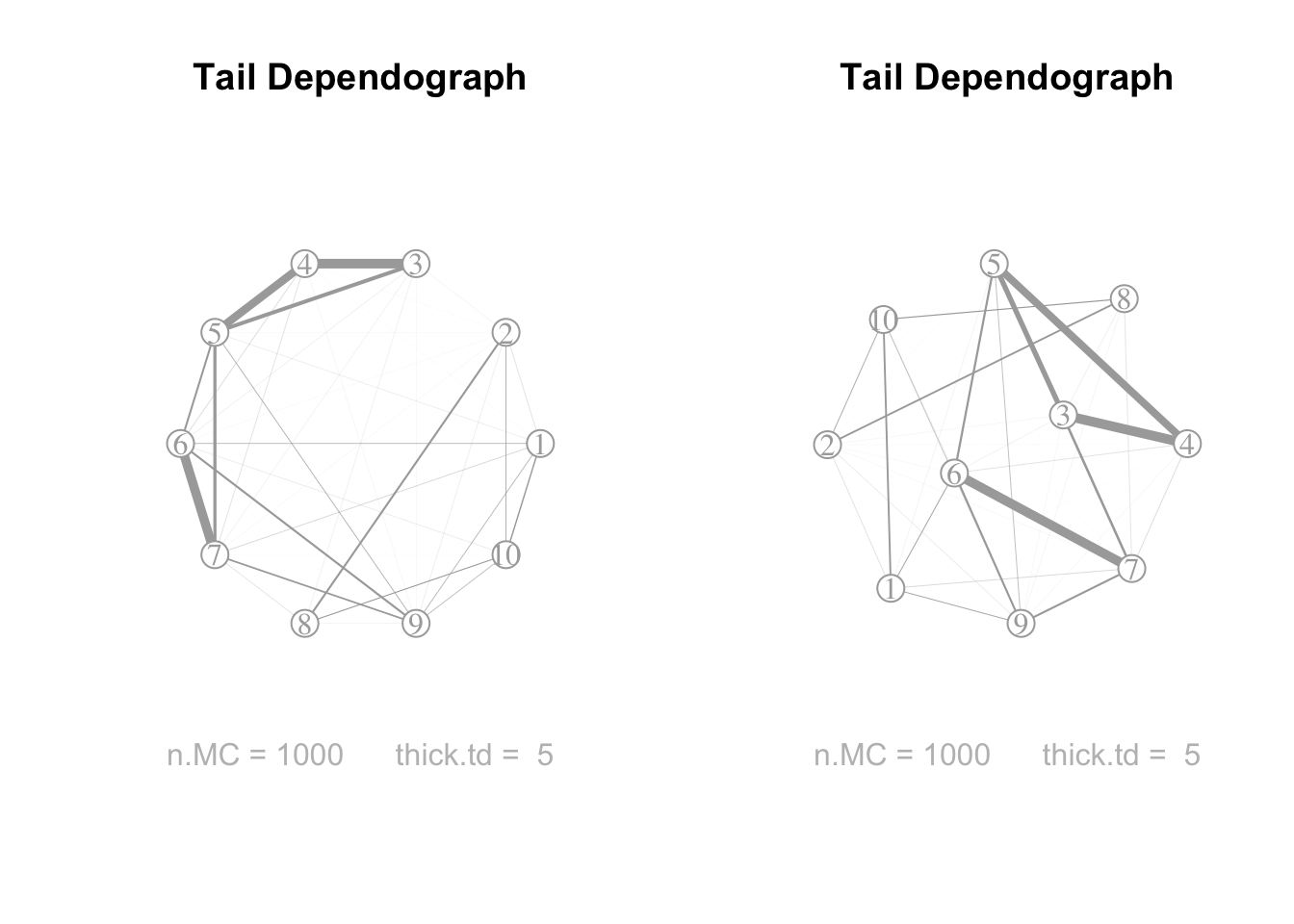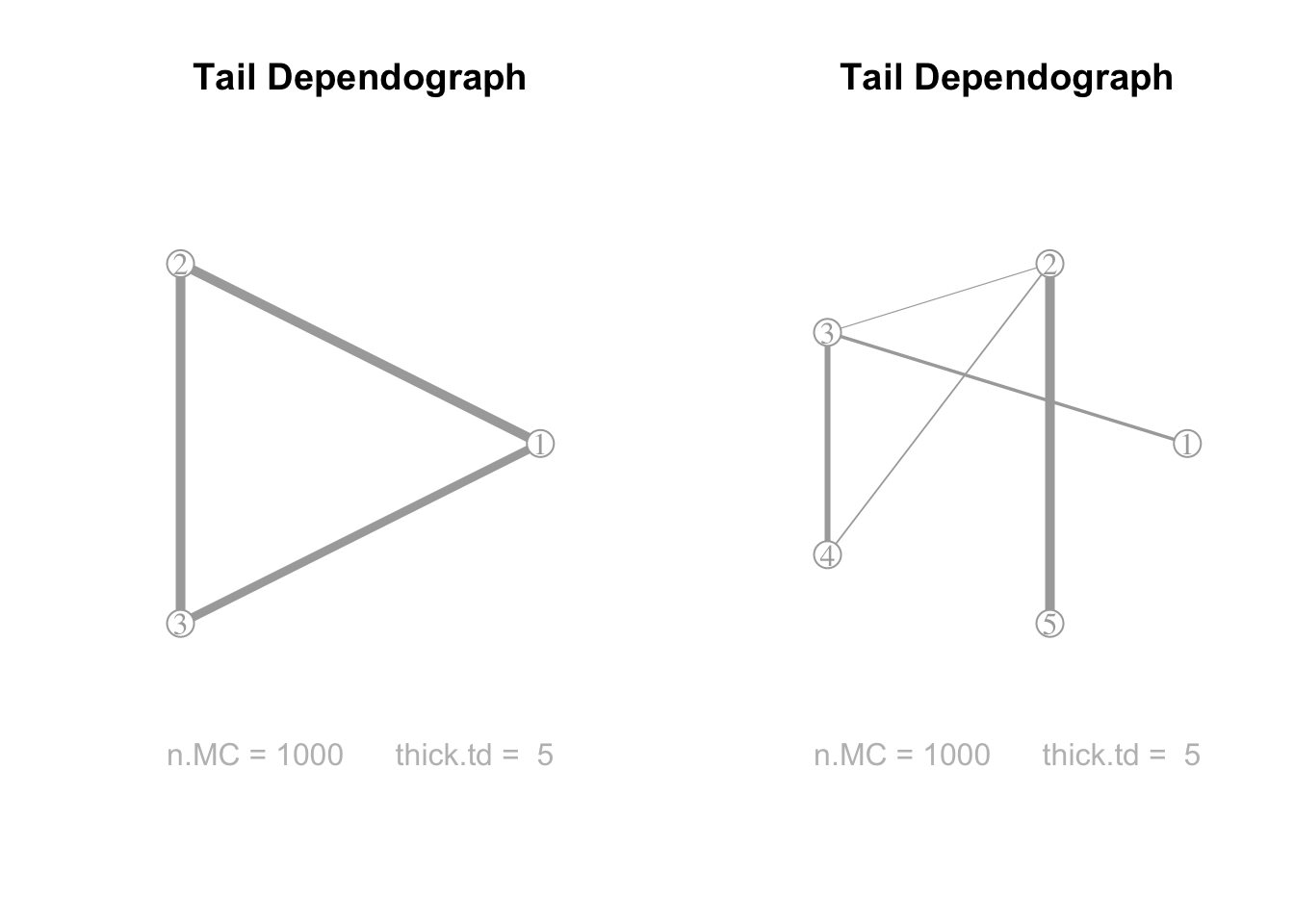# 1. Introduction & Installation

The name satdad is an acronym formed by the initials of Sensitivity Analysis Tools for Dependence and Asymptotic Dependence. The satdad R package provides tools for analyzing tail dependence in any sample or in particular theoretical models, namely Mevlog and ArchimaxMevlog. The package uses only theoretical and non parametric methods, without inference. Other tools and implementations will be added later to complete this first version. The primary goals of the package are to:

• Provide (a)symmetric multivariate extreme value models in any dimension Mevlog as some Archimax versions ArchimaxMevlog. Let us emphasize gen.ds, which generates easily tail dependence structure.

• Provide theoretical and empirical indices to order tail dependence. Let us emphasize tsic and tsicEmp which compute and estimate Tail Superset Importance Coefficients.

• Provide theoretical and empirical graphical methods to visualize tail dependence. Let us emphasize the theoretical and empirical tail dependograph plotted by graphs and graphsEmp.

The latest official release version can be obtained via

install.packages("satdad")

library(satdad)
## Loading required package: igraph
##
## Attaching package: 'igraph'
## The following objects are masked from 'package:stats':
##
##     decompose, spectrum
## The following object is masked from 'package:base':
##
##     union
## Loading required package: maps
## Loading required package: partitions
## Loading required package: graphicalExtremes

The mentioned R packages are used as dependencies as following:

• igraph functions graph, layout.fruchterman.reingold, and plot.igraph are called.

• The map function is imported from maps.

• The setparts function is imported from partitions.

• The danube dataset is extracted from graphicalExtremes for the illustrative session.

# 2. Theoretical modelling

Consider $${\bf X}=(X^{(1)},...,X^{(d)})$$ a $$d$$-variate random vector. Under standard Frechet margins, a multivariate extreme value (mev) random vector $$\bf X$$ has the cumulative distribution function

$\mathbb{P}\left(X^{(1)}\leq x_1,...,X^{(d)}\leq x_d\right)=\exp\left(-\ell\left(\frac{1}{x_1}, ..., \frac{1}{x_d}\right)\right)\;,$ for $$\ell$$ a stable tail dependence function. When restricting to (a)symmetric logistic dependence structure, such mev is called Mevlog in this package. It will also include mev with more general GEV margins.

Now, consider $${\bf U}=(U^{(1)},...,U^{(d)})$$ a $$d$$-variate random vector. Assume that the margins $$U^{(t)}$$, for $$t=1,...,d$$, follow the standard uniform distribution. The cumulative distribution function of $$\bf{U}$$ is then its copula function, $$\mathbb{P}(U^{(1)}\leq x_1, \ldots, U^{(d)}\leq x_d) = C(x_1,\ldots,x_d)$$. Assume that the copula function has the following form $C(x_1,\ldots,x_d)=\psi\left(\ell\left(\psi^{-1}(x_1),\ldots,\psi^{-1}(x_d)\right)\right)$ where $$\ell$$ is a stable tail dependence function and $$\psi$$ is the generator of a $$d$$-variate Archimedean copula. One can refer to Charpentier et al. (2014) for the description of $$\psi$$ and algorithms of simulation. Again, when $$\ell$$ is associated with a (a)symmetric logistic dependence structure, such model is called ArchimaxMevlog in this package.

## 2.1 Tail dependence structure

The tail structure of dependence is described by the stable tail dependence function $$\ell$$ defined above. In this package, it is saved in a ds object. The function gen.ds is used to generate these objects.

The multivariate asymmetric logistic model obtained through the option type = "alog". It generates a multivariate asymmetric logistic model, which has been first introduced by Tawn (1990). We have $\ell(x_1,\ldots,x_d)=\sum_{b\in B} (\sum_{i \in b} (\beta_{i,b}\, x_i)^{1/\alpha_b})^{\alpha_b}$ where $$B$$ is the power set of $$\{1,...,d\}$$ (or a strict subset of the power set), the dependence parameters $$\alpha_b$$ lie in $$(0,1]$$ and the collection of asymmetric weights $$\beta_{i,b}$$ are coefficients from $$[0,1]$$ satisfying $$\forall i \in \{1,\ldots,d\}, \sum_{b\in B: i \in b} \beta_{i,b}=1$$. Missing asymmetric weights $$\beta_{i,b}$$ are assumed to be zero.

The class ds is a list that consists of:

• the dimension $$d$$.

• the type (log or alog).

• the list sub that corresponds to $$B$$. When sub is provided, the same list of subsets is returned, eventually sorted. When sub = NULL then sub is a subset of the power set of $$\{1,...,d\}$$. When the option mnns is used, the latter integer indicates the cardinality of non singleton subsets in $$B$$.

• the dependence parameter dep = $$\alpha$$ or the vector of dependence parameters dep = $$\{\alpha_b, b \in B\}$$. When missing, these coefficients are obtained from independent standard uniform sampling.

• the list asy of asymmetric weights $$\beta_{i,b}$$ for $$b \in B$$ and $$i \in b$$. When missing, these coefficients are obtained from independent standard uniform sampling followed by a renormalization in order to satisfy the sum-to-one constraints.

Let us consider some examples.

## Construction of a ds object without using gen.ds
ds5 <- vector("list")
ds5$d <- 5 ds5$type <- "alog"
ds5$sub <- list(c(1,3),2:4,c(2,5)) ds5$asy <- list(c(1,.3),c(.5,1-.3,1), c(1-.5,1))
ds5$dep <- c(.2,.5,.3) For larger dimensions, defining a ds object can become tricky, and the use of gen.ds is very helpful. For example, a 10-dimensional asymmetric tail dependence structure can be randomly created as follows. ## Three constructions of ds object by using gen.ds # only d is given, sub, asy and dep are randomly sampled ds10 <- gen.ds(d = 10) # d and sub are given, asy and dep are randomly sampled ds10 <- gen.ds(d = 10, sub = list(1:2,1:7,3:5,7:10)) # d is given, mnns indicates the cardinality of non singleton subsets in B # sub, asy and dep are randomly sampled ds10 <- gen.ds(d = 10, mnns = 4) The symmetric case can be obtained through the type = "log" option in the gen.ds function, which yields a multivariate symmetric logistic model. This model is a well-known generalization of the bivariate extreme value logistic model introduced by Gumbel (1960). The parameter dep (with $$0 < {\rm dep} \leq 1$$) is the only parameter needed to write the following equation $\ell(x_1,\ldots,x_d) = ( \sum_{i=1}^d x_i^{1/{\rm dep}} )^{\rm dep}.$ If the parameter dep is missing, the function gen.ds will randomly generate its value from a standard uniform distribution. For example, to obtain a 3-dimensional symmetric tail dependence structure with a randomly generated dependence parameter, you can use the following code ds3 <- gen.ds(d = 3, type = "log") ds3$dep
##  0.3197499

If you know the value of the dependence parameter, you can specify it by setting ds3$dep <- .3, or by using the dep argument directly ds3 <- gen.ds(d = 3, type = "log", dep = .3) ## 2.2 Sampling models As mentioned at the beginning of the previous section, the satdad package studies both the Mevlog and ArchimaxMevlog theoretical models. Samples of the MEV random vector with logistic dependence structures can be obtained via the rMevlog function using Algorithms 2.1 and 2.2 in Stephenson(2003). n <- 1000 sample.frechet <- rMevlog(n, ds5) # standard Frechet margins loc <- runif(5) scale <- runif(5, 1, 2) shape <- runif(5, -1, 1) mar.gev <- cbind(loc, scale, shape) sample.gev <- rMevlog(n, ds5, mar = mar.gev) # GEV margins all distinct sample.samegev <- rMevlog(n, ds5, mar = c(-1,0.1,1)) # Gumbel margins In addition, the package provides functions for computing the stable tail dependence function (ellMevlog), cumulative distribution function (pMevlog), and probability density function (dMevlog) of the Mevlog distribution. The following are examples of commands for these functions, but without evaluation. x5 <- runif(5) ellMevlog(x5, ds5) pMevlog(x5, ds5) # cdf under standard Frechet margins pMevlog(x5, ds5, mar = c(1,1,0)) # cdf under standard Gumbel margins dMevlog(x5, ds5) # pdf under standard Frechet margins In addition to multivariate extreme value logistic models, referred to as Mevlog, the satdad package provides some particular cases of ArchimaxMevlog. We follow here Algorithm 4.1 of p. 124 in Charpentier et al. (2014). Let $$\psi$$ defined by $$\psi(x)=\int_0^\infty \exp(-x t) dF_V(t)$$, where $$F_V$$ is the cumulative distribution function of a positive random variable. We define the random vector $$(U_1,...,U_d)$$ as $$U_i=\psi(-\log(Y_i)/V)$$ where • $$Z$$ has a multivariate extreme value distribution with stable tail dependence function $$\ell$$ ; here $$Z$$ has standard Frechet margins, • $$(Y_1,...,Y_d)=(\exp(-1/Z_1),...,\exp(-1/Z_d))$$ is the margin transform of $$\bf Z$$ so that $$\bf Y$$ is sampled from the extreme value copula associated with $$\ell$$, • $$V$$ has the distribution function $$F_V$$, • $$Y$$ and $$V$$ are independent. Then, $$\bf U$$ is sampled from the Archimax copula $C(x_1,\ldots,x_d) = \psi(\ell(\psi^{-1}(x_1),\ldots,\psi^{-1}(x_d)))\;.$ The package provides ArchimaxMevlog realizations of random vectors $$\bf U$$. The cases covered by the satdad package are as follows: $$\psi$$ is one among three types: • $$\psi(t)=\exp(-t)$$ ; set dist = "ext". • $$\psi(t)=\dfrac{{\rm lambda}}{t+{\rm lambda}}$$ ; set dist = "exp" and dist.param = lambda. • $$\psi(t)=\dfrac{1}{(t+{\rm scale})^{\rm shape}}$$ ; set dist = "gamma" and dist.param = c(shape, scale). $$\ell$$ is the stable tail dependence function (stdf) associated with (a)symmetric logistic extreme value models. ArchimaxMevlog samples are obtained via n <- 1000 sample.ext <- rArchimaxMevlog(n, ds5, dist = "ext") lambda <- runif(1, 1, 2) sample.exp <- rArchimaxMevlog(n, ds5, dist = "exp", dist.param = lambda) shape <- runif(1, 1, 2) scale <- runif(1, 1, 2) sample.gamma <- rArchimaxMevlog(n, ds5, dist = "gamma", dist.param = c(shape, scale)) The satdadpackage provides functions for computing $$\ell$$ $$C$$, $$\psi$$, and $$\psi^{-1}$$ for ArchimaxMevlog models. Specifically, ellArchimaxMevlog, copArchimaxMevlog, psiArchimaxMevlog and psiinvArchimaxMevlog can be used. x <- runif(5) ellMevlog(x, ds5) ##  2.702487 ellArchimaxMevlog(x, ds5) ##  2.702487 copArchimaxMevlog(x, ds5, dist = "ext") ##  0.3507565 copArchimaxMevlog(x, ds5, dist = "exp", dist.param = lambda) ##  0.3959051 copArchimaxMevlog(x, ds5, dist = "gamma", dist.param = c(shape, scale)) ##  0.3841986 ## 2.3 Measures and plots of the tail dependence The tail dependence is completely characterized by the stdf $$\ell$$, or equivalently by the ds object in this package. Summaries and graphical tools are obviously appreciated. Well known extremal coefficients (ec), introduced by Tiago de Oliveira, J. (1962/63) and Smith (1990), are available in satdad. However, the focus is on the tail superset importance coefficients, which were introduced in Mercadier and Roustant (2019) and upper bounded in Mercadier and Ressel (2021). We believe that they also offer an interesting perspective on the description of the structure of the stable tail dependence function. The theoretical functional decomposition of the variance of the stdf $$\ell$$ consists in writing $D(\ell) = \sum_{I \subseteq \{1,...,d\}} D_I(\ell)$ where $$D_I(\ell)$$ measures the variance of $$\ell_I(U_I)$$ the term associated with subset $$I$$ in the Hoeffding-Sobol decomposition of $$\ell$$ ; note that $$U_I$$ represents a random vector with independent standard uniform entries. Fixing a subset of components $$I$$, the theoretical tail superset importance coefficient (tsic) is defined as $\Upsilon_I(\ell)=\sum_{J \supseteq I} D_J(\ell)\;.$ An integral representation of the superset importance coefficient is provided by Formula (9) of Liu and Owen (2006). See also Mercadier and Roustant (2019) for its use in the extreme value context. Thus, the tsic here is the value of $$\Upsilon_I(\ell)$$ obtained by Monte Carlo methods from the integral formula (3) in Mercadier and Roustant (2019). res.tsic5 <- tsic(ds5) as.character(res.tsic5$subsets)
##   "1:2"     "c(1, 3)" "c(1, 4)" "c(1, 5)" "2:3"     "c(2, 4)" "c(2, 5)"
##   "3:4"     "c(3, 5)" "4:5"
res.tsic5\$tsic
##   1.320186e-32 7.768925e-04 1.393141e-32 9.174360e-33 1.591981e-04
##   3.699052e-04 1.729641e-03 9.495182e-04 8.504907e-33 7.775364e-33

The graphs function in satdad implements the methodology introduced in Mercadier and Roustant (2019). The default option which = taildependograph draws the PAIRWISE tsic in a graphical representation called the tail dependograph. The command is as follows.

oldpar <- par(mfrow=c(1,2))
graphs(ds10) # (left) the nodes are plotted on an invisible circle
graphs(ds10, random = TRUE) # (right) the position of the nodes are randompar(oldpar)
oldpar <- par(mfrow=c(1,2))
graphs(ds3) # (left) the symmetric structure
graphs(ds5) # (right) the asymmetric structure contructed "manualy"par(oldpar)

A theoretical upper bound for tsic $$\Upsilon_I(\ell)$$ is given by Theorem 2 in Mercadier and Ressel (2021) which states that $\Upsilon_I(\ell)\leq \frac{2(|I|!)^2}{(2|I|+2)!}$ for any stdf $$\ell$$. This allows for meaningful comparison of these indices, regardless of the cardinality of $$I$$, using the expression $\dfrac{\Upsilon_I(\ell)}{D(\ell)}\times \frac{(2|I|+2)!}{2(|I|!)^2}\;.$ The option sobol = TRUE provides the renormalization by $$D(\ell)$$, while norm = TRUE multiplies by the inverse of the upper bound. The Cleveland dot plot is a useful tool to globally compare these coefficients.

plotClev(ds5)